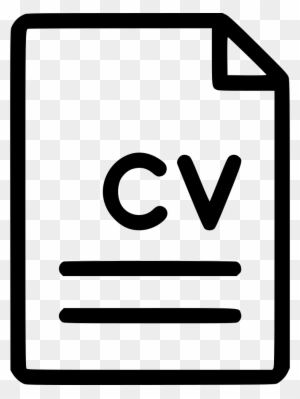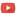KE8QZC | SFW | TSWSyllabus: [pdf] [tex]
Code repository
SWISH

Previous course materials
Spring 2017 (Fairmont)
Exams
Exam 1 [pdf] [tex]
Exam 2 [pdf] [tex]
Exam 3 [pdf] [tex]

Homework
Homework 1 (due 23 January) (solution: [pdf] [tex]): [pdf] [tex]
Homework 2 (due 30 January) (solution: [pdf] [tex]): #1.1, 1.3, 1.4, 1.5, and the following Prolog exercise: [pdf] [tex]
Homework 3 (due 15 February) (solution: [pdf] [tex]): #1.6, 1.9, 1.10, 1.12 and the following two problems:
Problem A: Write a formal proof that shows $\{P,P \rightarrow Q\} \vdash Q$.
Problem B: Write a formal proof that shows $\{P \rightarrow Q, Q \rightarrow R, S \vee \neg R, P\} \vdash S$.
Homework 4 (due 20 February) (solution): #1.7, 1.11, 1.18, 1.24, 1.27(a)
Homework 5 (due 1 March) (solution): #1.8, 1.20 and the following Prolog stuff: [pdf] [tex]
Homework 6 (due 8 March) (solution): #2.1, 2.2, 2.7
Homework 7 (due 20 March) (solution): #2.5(a), 2.8, 2.9, 2.11
Homework 8 (due 5 April) (solution: [pdf] [tex]): [pdf] [tex]
Homework 9 (due 12 April) (solution: [pdf] [tex]): [pdf] [tex]
Homework 10 (due 17 April) (solution): [pdf] [tex]
Homework 11 (due by last day of finals) (solution): [pdf]

In-class work
Quiz 1 (solution)
Quiz 2 (solution)
Quiz 3 (solution)
Quiz 4 (solution)
Quiz 5 (solution)

Notes
18 January 2018 -- notes on relations: [pdf] [tex]
15 February 2018 -- is $A \wedge (B \vee B)$ equivalent to a Horn formula?: [answer]
18 February 2018 -- induction example (problem 1.27(b)): [solution]
22 February 2018 -- full list of rules for propositional logic proof system: [pdf] [tex]
Set theory proofs from class
Monoid theory example
Slides from last week of class